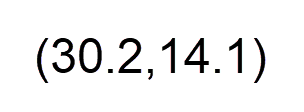﻿ Bivariate Data

# Bivariate Data

Top  Previous  Next
 You can include bivariate data information in data parameters using the following format:You can list these points out in a data parameter, with the values separated by commas (or semicolons if you use a comma as a decimal point) OR no separator at all.   \$b=data((11,12)(15,19)(21,28)(13.2,19.4),(8,16))   There are a number of statistical commands that can work with bivariate data. In particular, regslope, regintercept and regr.   You can access individual entries in the data parameter using references. For example, if you type \$b, the system will return  (21,28).   You can access the x value and y value components of a point using the xvalue and yvalue commands in the calculator.   {xvalue(\$b)}                will return 21 {yvalue(\$b)}                will return 28